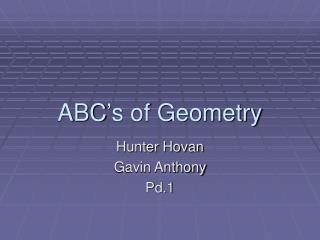DownloadDownload PresentationABC’s of Geometry

# ABC’s of Geometry

Download Presentation## ABC’s of Geometry

- - - - - - - - - - - - - - - - - - - - - - - - - - - E N D - - - - - - - - - - - - - - - - - - - - - - - - - - -
##### Presentation Transcript

1. ABC’s of Geometry Hunter Hovan Gavin Anthony Pd.1

2. A=Angle • The union of 2 rays that have the same endpoint

3. B=Base • The surface that a solid stands on

4. C=Circumference • The perimeter of a circle

5. D=Decagon • A ten-sided polygon

6. E=Equilateral Triangle • Equal in length on all sides

7. F=Flip • Make a mirror image of one shape or object

8. G=Graph • A diagram of values • Usually shown with line or bars

9. H=Hexagon • A six sided polygon

10. I=Isosceles Triangle • A triangle with two equal sides

11. J=AdJacent Angle • Two angles that share a common Vertex and don’t overlap

12. K=Kite • A quadrilateral that has two distinct pairs of consecutive equilateral sides

13. L=Line • Is straight (no line) • Has no thickness

14. M=Measurement • The process of assigning a number to a physical property.

15. N=Number Line • A line that represent the set of numbers

16. O=Obtuse Angle • An angle that has measure more than 90° and less than 180°

17. P=Pi • The ratio of the circumference of a circle to its diameter • π = 3.141592653589793

18. Q=Quadrilateral • A polygon with four sides.

19. R=Rectangle • A box shape on a plane.

20. S=Square • A rectangle with all four sides of equal length.

21. T=Triangle • A polygon with three sides.

22. U=Undecagon • A polygon with eleven sides.

23. V=Vertex • A corner point of a geometric figure.

24. S=SkeW Lines • Lines in three dimensional space that do not intersect and are not parallel

25. X=X-Axis • The line on a graph that runs horizontally (left-right) through zero.

26. Y=Y-Axis • The line on a graph that runs vertically (up-down) through zero.

27. Z=Z-Axis • The third line in a 3-dimensional coordinate system.

28. ¡THE END!